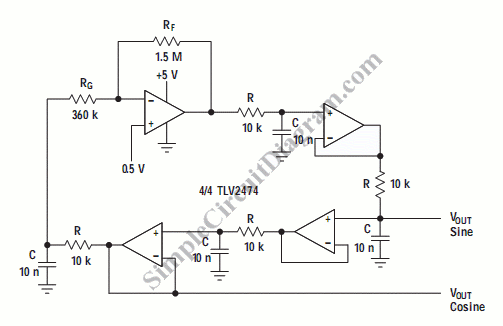# Bubba Oscillator

One example of the  phase shift oscillator is Bubba oscillator. This oscillator gets 45 degree phase shift for each section from quad op-amp package. The result is excellent d(phase)/d(t) to obtain very low frequency drift. The circuit is shown below:45 degree phase shift is obtained from Each RC section. The alternating section generate a low impedance quadrature output. The differences of each of the op amp output is 45 degrees. This circuit has a frequency about 1.76kHz. RF and RG sets the gain and must be  slightly higher than 4. The lowest distortion  output is given by the junction between R and RG.  If we need very low distortion on all outputs, then the gain should be distributed equally on all op-amps. [Schematic diagram source:  Texas Instrument Analog Applications Journal]